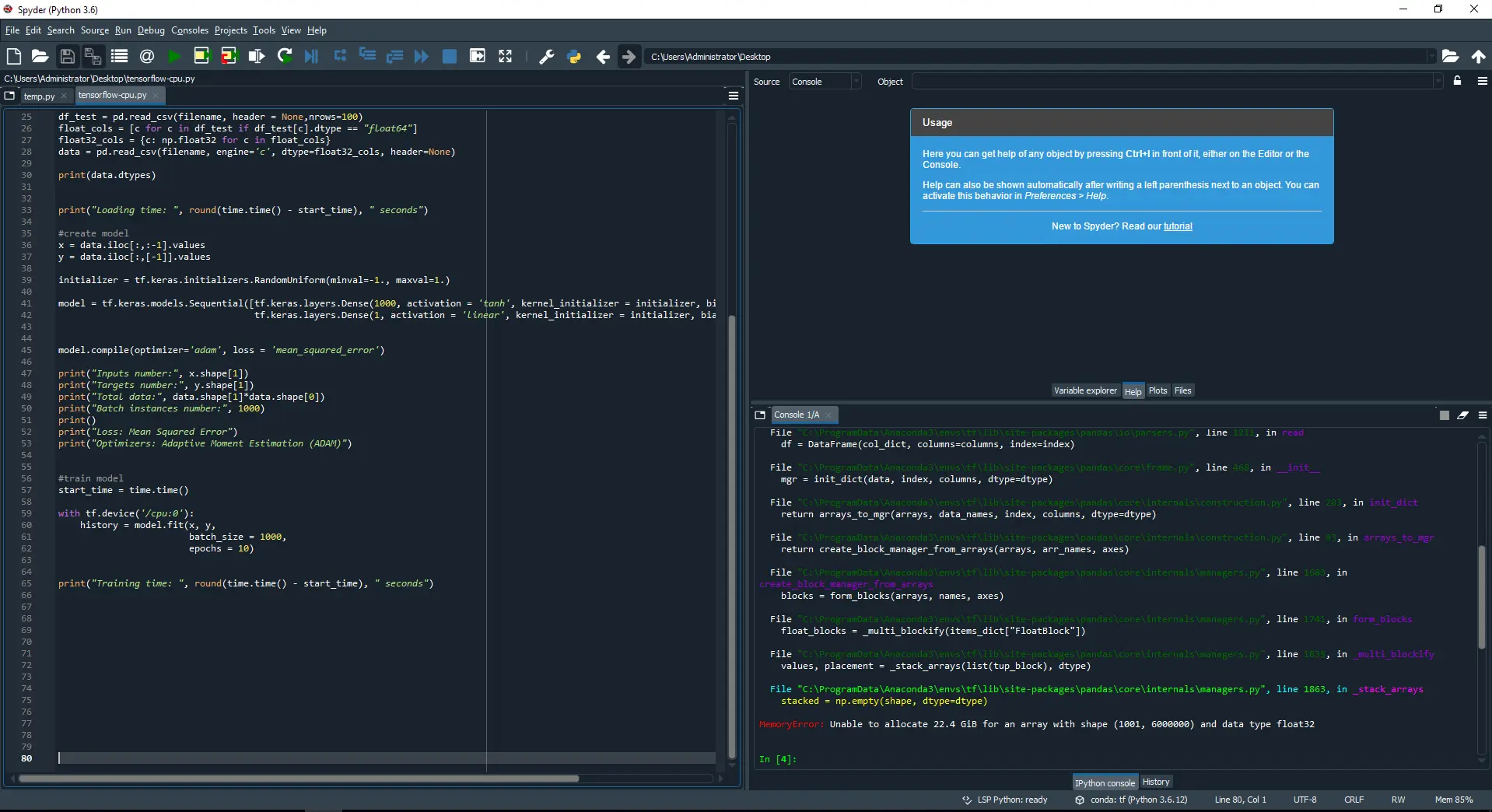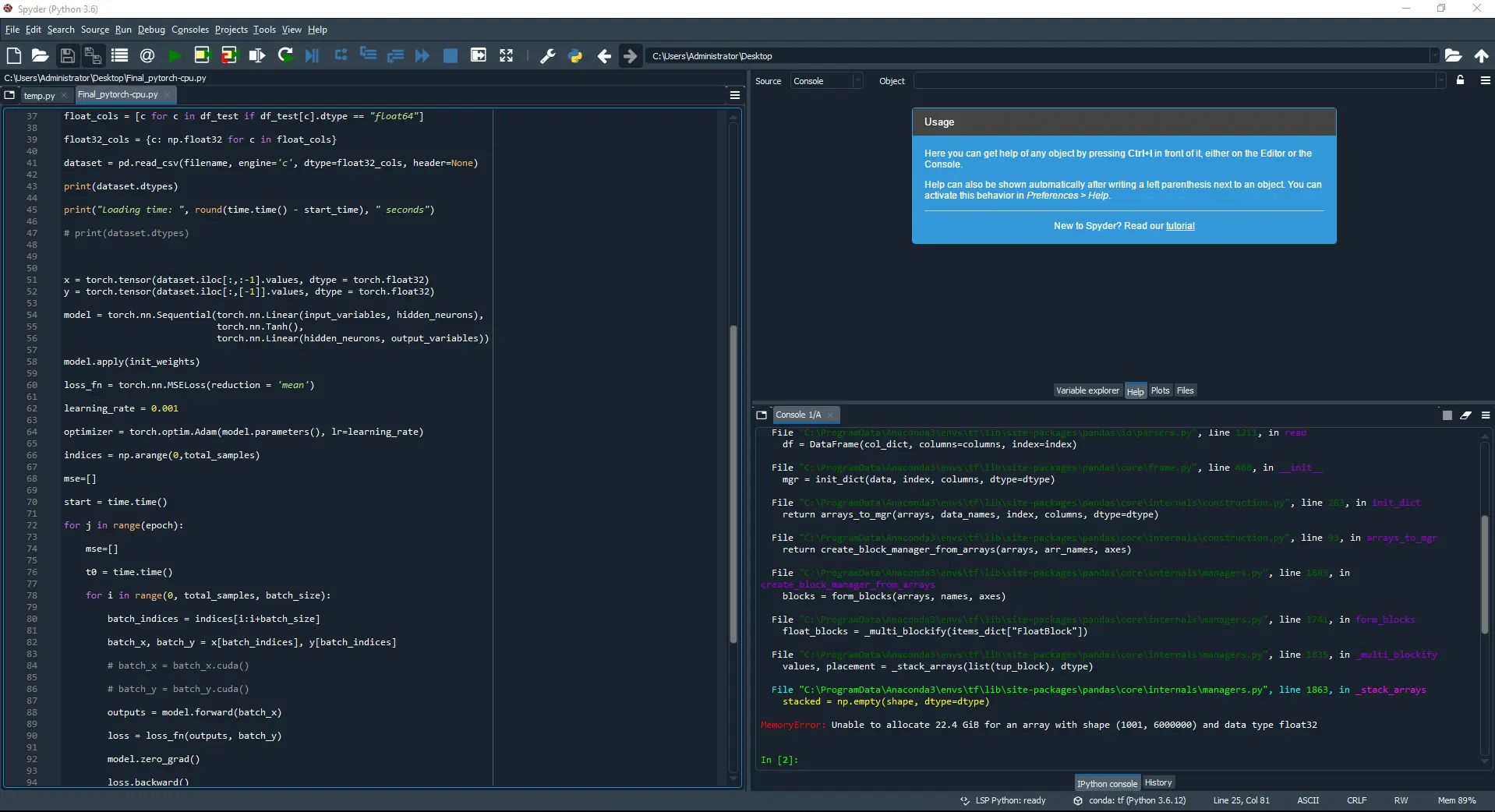﻿ Capacity comparison TensorFlow vs PyTorch vs Neural-Designer

# Capacity comparison: TensorFlow vs PyTorch vs Neural DesignerCapacity means the maximum amount of data a computer program can analyze in data science and machine learning platforms.

In this post, we compare the load capacity of three machine learning platforms: TensorFlow, PyTorch and Neural Designer for an approximation benchmark. These platforms are developed by Google, Facebook and Artelnics, respectively.

As we will see, Neural Designer is able to load a dataset x1.8 larger than TensorFlow and PyTorch.

In this article, we provide all the steps that you need to reproduce the results using the free trial of Neural Designer.

Contents:

## Introduction

The maximum amount of data a tool can analyze depends on different factors. Some of the most important ones are the programming language in which it is written and how the memory usage works internally.

The following table summarizes the technical features of these tools that might impact their memory usage.

TensorFlow PyTorch Neural Designer
Written in C++, CUDA, Python C++, CUDA, Python C++, CUDA
Interface Python Python Graphical User Interface

Even though C++ is at the core of the three platforms, their interfaces are different. The most common use of TensorFlow and PyTorch is through a Python API. On the other hand, Neural Designer uses a C++ GUI.

As we will see, an application with a Python interface results in higher memory consumption, which means a lower capacity to load the data.

## Benchmark application

To test the capacity of the three platforms, we will try to load different Rosenbrock datafiles, fixing the number of variables and changing the number of samples. The following table shows the correspondence between the size and the number of Rosenbrock samples.

Filename Floating points number (x109) Samples number Size (Gb)
Rosenbrock_1000_variables_1000000_samples.csv 1 1000000 22
Rosenbrock_1000_variables_2000000_samples.csv 2 2000000 44
Rosenbrock_1000_variables_3000000_samples.csv 3 3000000 65
Rosenbrock_1000_variables_4000000_samples.csv 4 4000000 86
Rosenbrock_1000_variables_5000000_samples.csv 5 5000000 107
Rosenbrock_1000_variables_6000000_samples.csv 6 6000000 128
Rosenbrock_1000_variables_7000000_samples.csv 7 7000000 149
Rosenbrock_1000_variables_8000000_samples.csv 8 8000000 171
Rosenbrock_1000_variables_9000000_samples.csv 9 9000000 192
Rosenbrock_1000_variables_10000000_samples.csv 10 10000000 213

To create these files check this article: The Rosenbrock Dataset Suite for benchmarking approximation algorithms and platforms.

The number of samples is the only parameter we change to perform the comparison tests. The other parameters are constant. The next picture shows the benchmarks set up.

Data setBenchmark: Rosenbrock Inputs number: 1000 Targets number: 1 Samples number: Table Layers number: 2 Layer 1: -Type: Perceptron (Dense) -Inputs number: 1000 -Neurons number: 1000 -Activation function: Hyperbolic tangent (tanh) Layer 2: -Type: Perceptron (Dense) -Inputs number: 1000 -Neurons number: 1 -Activation function: Linear Initialization: Random uniform [-1,1] Loss index: -Error: Mean Squared Error (MSE) -Regularization: None Optimization algorithm: -Algorithm: Adaptive Moment Estimation (Adam) -Batch size: 1000 -Maximum epochs: 1000

We run the above benchmark for each platform (TensorFlow, PyTorch, and Neural Designer), increasing samples until the memory crashes. We consider a successful test if it can load the CSV file and train the neural network.

## Reference computer

The next step involves choosing the computer to train the neural networks with TensorFlow, PyTorch, and Neural Designer. For a capacity test, the most crucial feature of the computer is its memory.

We have made all calculations on an Amazon Web Services instance (AWS). In particular, we have chosen the r5.large. so that you can reproduce the results easily. The next table lists some basic information about the computer used here.

Operating system Windows 10 Enterprise
Processor Intel(R) Xeon(R) Platinum 8259CL CPU @ 2.50GHz
Installed memory (RAM): 16.0 GB
System type: 64-bit Operating system, x64 based processor

Once the computer is chosen, we install TensorFlow (2.1.0), PyTorch (1.7.0) and Neural Designer(5.0.0) on it.

## Results

The last step is to run the benchmark application using TensorFlow, PyTorch, and Neural Designer. Then, we compare the capacity results provided by those platforms.

The following table shows whether or not each platform can load the different data files. The blue check means that the platform can load it and the orange cross means that it is not able.

Floating points
number (x109)
TensorFlow PyTorch Neural Designer
12345678910As we can see, the maximum capacity of both TensorFlow and PyTorch is 5 x 109 data and the maximum capacity of Neural Designer is 9 x 109 data.

These results can also be depicted graphically.From these results, we can conclude that Neural Designer is able to load a dataset x1.8 larger than TensorFlow and PyTorch.

The following picture shows that Neural Designer can train a neural network with 9 billion data points in a 16GB RAM computer.The following picture shows how TensorFlow runs out of memory when trying to load a data file containing 6 billion data.As we can see, when the external module Python pandas in TensorFlow tries to load 6 billion data, the platform crashes due to lack of RAM. TensorFlow's maximum capacity is 5 billion data.

The following picture shows how PyTorch also runs out of memory when loading a data file containing 6 billion data.Again, when the external module Python pandas in PyTorch tries to load 6 billion data, it crashes. PyTorch's maximum capacity is 5 billion data.

## Conclusions

The maximum capacity in both TensorFlow and PyTorch is 5 billion data, and the maximum capacity in Neural Designer is 9 billion.

This difference is because TensorFlow and PyTorch use an external module (Python pandas) to load data. In contrast, Neural Designer uses its function to load data, which offers an advantage.

Indeed, Python is a high-level programming language. However, this causes a lower capacity for Python tools to load data.